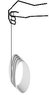# Rotational dynamics question 2

squintyeyes
how do you do part c, d, and e

A string is wrapped several times around the rim of a small hoop of radius 0.0690 m and a mass 0.152 kg. The situation is sketched in the figure below.

If the free end of the string is held in place and the hoop is released from rest, calculate the following quantities:

(a) the magnitude of the acceleration of the hoop's center of mass
4.9 m/s2

(b) the tension in the string while the hoop descends and the string unwinds
0.745 N

(c) the time it takes the hoop to descend 0.600 m
_________ s

(d) the speed of the hoop's center of mass after it's descended 0.600 m
__________m/s

(e) the angular speed of the rotating hoop after it's descended 0.600 m

Attempt
Σ Forces in x direction = ma
mg - Tension = ma
mg - T= ma

Σ Torque = Iα
Iα = Tr
(mr^2)(a/r)= Tr
T= ma

mg - T = ma
mg- ma = ma
mg = 2ma
g = 2a
a = g/2 = 9.8/2= 4.9

T = ma
= (0.152)(4.9)
= 0.7448

#### Attachments

•Rotational Dynamics 2.jpg
3.4 KB · Views: 374

Mentor
how do you do part c, d, and e
You found the acceleration; use kinematics to solve those parts of the problem.

squintyeyes
so for part c, would i just do

Δy = vt + (1/2)at^2
0.6 = 0 + (1/2) (4.9) t^2
t^2 = 0.244897
t = 0.495 s

then d is found by

final velocity = inital velocity + at
= 0 + (4.9)(0.495)
= 2.426 m/s

Lastly e is found by

σ = v/r
= 2.426/0.069